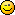<![CDATA[Math Is Fun Forum / Whats the perimeter?]]> 2007-03-10T16:09:01Z FluxBB https://www.mathisfunforum.com/viewtopic.php?id=6357 <![CDATA[Re: Whats the perimeter?]]> Refer to Toasts diagram. The angle θ in the diagram is π⁄3 radians. Twice that is 2π⁄3 radians. So the length of the arc subtending this angle (one vertical half of the lime curve in Toasts diagram) is (2π⁄3)r. (Get it? If not, read up on the definition of the radian.)

∴ The perimeter of C (i.e. length of the lime curve in Toasts diagram) is 2×(2π⁄3)r = (2π⁄3)d = . Thats my answer (same as Toasts).]]>
https://www.mathisfunforum.com/profile.php?id=6777 2007-03-10T16:09:01Z https://www.mathisfunforum.com/viewtopic.php?pid=63134#p63134
<![CDATA[Re: Whats the perimeter?]]> Ok, lemme have a go at this *IT MAY NOT BE CORRECT*

I hope I have interpreted this correctly and the coloured part of the diagram is the length you've asked me to find.

You might wanna look at the attached diagram first:

Double theta to find the angle needed for one half of the total perimeter:

Then, make it a fraction of the whole circle then multiply by pi and the diameter :

As the two halves of the perimeter are in fact equal, simply multiply this by 2 too, and you will get the total perimeter:

And, as

and cos(60) = 1/2, therefore arccos(1/2) = 60. Simplify the rest and you will get

(I am always amazed at how you can reduce something so complex to something so simple and workable)

Plug in 1 and you get Approximately 2.09439510239.

Am I right?]]>
https://www.mathisfunforum.com/profile.php?id=5267 2007-03-10T15:11:18Z https://www.mathisfunforum.com/viewtopic.php?pid=63128#p63128
<![CDATA[Whats the perimeter?]]> I have 2 circles (let us say A and B) of equal diameter, and they overlap each other by passing through the center point of each other (ie the path of one circle passes through the center point of the other circle). Let us name the common region as C, now what is the perimeter of the C? I am not sure if C is a circle.

Thanks!

]]>
https://www.mathisfunforum.com/profile.php?id=5477 2007-03-10T14:40:55Z https://www.mathisfunforum.com/viewtopic.php?pid=63123#p63123